# Shear bending stresses in beams

In this tutorial we investigate shear stresses induced in a beam by bending.

Analysis of shear bending stress is more complex than normal bending stress and this tutorial provides a basic introduction valid for beams of rectangular cross-section with height significantly greater than breadth.

The diagram below illustrates the origin of shear stress in bending.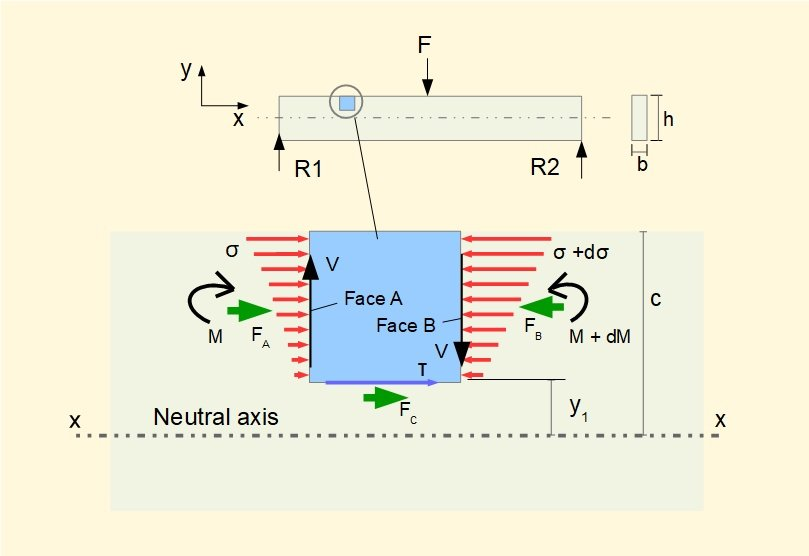The diagram shows a beam of rectangular cross-section with height h and width b.

A small element of the beam height y1 above the neutral axis x-x with vertical faces A and B is subject to shearing forces V.  The bending moment M varies along the beam and from our previous study we know: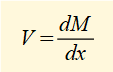Also from our study of normal bending stress we know σ is directly proportional to M.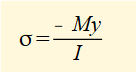It follows that the mean normal stress on face B must be greater than on face A and thus the net the force FB on face B must be greater than the net force FA on face A.

For the element to be in equilibrium the sum of horizontal forces must equal zero.  Thus a horizontal force FC is exerted on the bottom face of the element resulting in a shear stress τ on this face.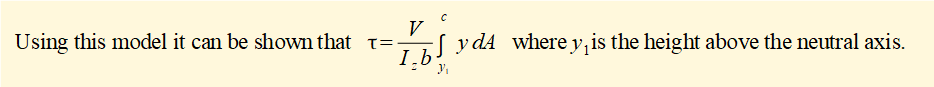Note that Iz is the second moment of area of the entire cross-section about the neutral z axis.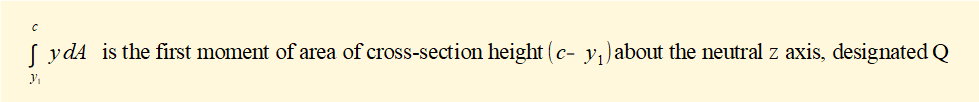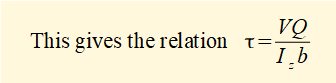We know that the shear stress on a plane in a biaxial element is accompanied by shear stresses of equal magnitude on the other three orthogonal planes with directions such that the element is in equilibrium.  Hence a shear stress of the same magnitude exists on the other faces of the element as shown below.

In this model it was assumed that V has the same value on both vertical faces of the element, which is true for beams with point loading.  For distributed loads, V is not constant but when the length of the element → 0 the expression above for τ is valid.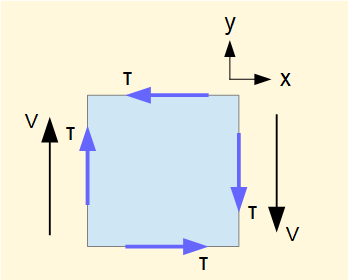We now examine the distribution of shear stress on the y-z plane in the case of the rectangular section below.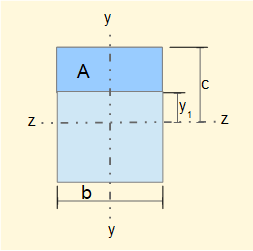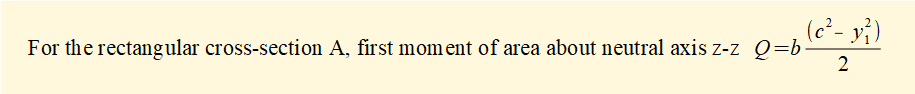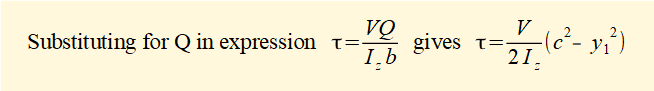Distribution of τ across the section for values of y1 from -c to +c is a quadratic function as shown below.  τmax occurs at the neutral axis where y1 = 0 and τ = 0 where y1 = ±c.  Note that locations of minimum and maximum shear bending stresses are the reverse of normal bending stresses.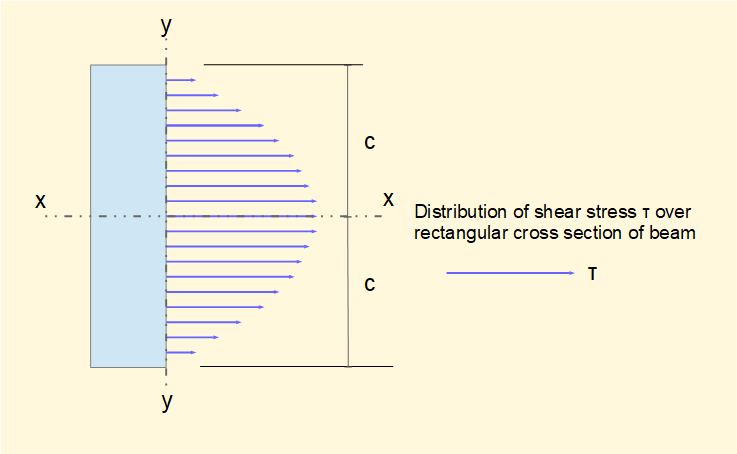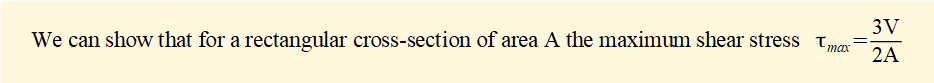Compare this value with the assumption of uniformly distributed shear stress over the cross-section for shearing force V giving: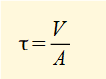i.e.  τmax is greater by a factor of 3/2.  Similar factors for solid circular and hollow circular sections are 4/3 and 2 respectively.

I welcome feedback at:

### Tutorials - mechanical vibrations

###### Forced vibrations with damping

Alistair's tutorials 2019